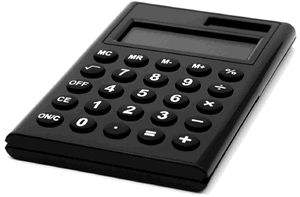# Calculator Fun

Use your own calculator, or the Full Precision Calculator for many more digits.

Many of these seem like magic, but there is always a reason ... see if you can discover it!## Seven

Try this in a calculator: 1/7

We get 0.142857142857...

2/7 gets 0.285714285714...

3/7 gets 0.428571428571...

In each case it is the same digits "142857" repeating over and over again, justy starting at a different spot.

## Nine

Try this in a calculator: 1/9

We get 0.11111111...

2/9 gets 0.22222222...

8/9 gets 0.88888888...

9/9 gets ?

## Nines Squared

Now try 1/9^2 (1 divided by 9 squared which equals 1/81)

We get 0.0123456790123...

All the digits from 0 to 9 repeat over and over again, but '8' is missing!

Now try 1/99^2 (1 divided by 99 squared which equals 1/9801)

We get 0.0001020304050607080910111213141516171819 etc

Do you see the decimals are 00,01,02,03,... (but '98' is actually missing)

Now try 1/999^2 (=1/998001)

We get 0.000001002003004005006007008009010011012 etc

the decimals are 000,001,002,003,... (but '998' is actually missing)

## Eighty Nine

1/89 = 0.011235955056179775280898876404494382022471910112...

The first few digits (0,1,1,2,3,5) are the Fibonacci sequence

In a way they all are, except multiple digit numbers (13, 21, etc) overlap, like this:

 0.0 0.01 0.001 0.0002 0.00003 0.000005 0.0000008 0.00000013 0.000000021 ... etc ... 0.011235955056179775...  =  1/89

## Seven Again

Try this in a calculator: 1/7^2

We get 0.02040816326530...

Notice how every two digits double each time: 2, 4, 8, 16, 32, 64 (well 65, because it is being overwritten with the next number 128 similar to the above)

Now try 1/49999 on the Full Precision Calculator to see what you get.

## Ends With Seven Magic

Type in a random 3 digit number twice

Now divide by 11: 638638 / 11 = 58058

Now divide by 13: 58058 / 13 = 4466

Then divide by the original number: 4466 / 638 = 7

You will always end with 7

## Ends With Seventy Three Magic

Type in a random 4 digit number twice

Now divide by 137: 63856385 / 137 = 466105

Then divide by the original number: 466105 / 6385 = 73

You will always end with 73

## Guess the Number

Ask someone to choose a number between 1 and 9

Have them multiply their number by 12345679 (no 8 OK) and they will have a big number like 86419753. Now ask them to give you the calculator.

You then multply that number by 9 (let them see you only press a few buttons), and you will see a number like 777777777, which means their chosen number was 7

## Guess The Numbers

Get a friend to choose two numbers between 1 and 9

Get them to multiply one of them by 5, then add 7, then multiply all of that by 2

Now get them to add their other number, and announce the result.

You can now subtract 14 and the two digit number remaining is the two original numbers next to each other

Example: 4 and 9

They calculate: 4 x 5 = 20, + 7 = 27, x 2 = 54, + 9 = 63.

You subtract 14 from that and get 49. You can then say "your original numbers were 4 and 9".

## Calculator WordsDo the calculation, then rotate the display 180°:

• 206 × 3 BIG
• 454 × 17 BILL
• 13 × 26 BEE
• 1 − 0.98 ZOO
• 101 × 8 BOB
• 1 − 0.2266 HELLO
• 333 + 204 LES
• 101 × 7 LOL
• 499 × 155 SHELL
• 115469 × 5 SHELLS
• 819 × 7 EELS
• 5555 + 82 LEGS
• 0.0113 × 7 IGLOO
• 73 × 106 BELL
• 0.18 + 0.19 LEO
• 68 × 81 BOSS
• 1102 × 7HILL
• 5001 × 7LOOSE
• 27689 × 2BLESS
• 1262 × 28GEESE
• 10238 × 37 GOBBLE